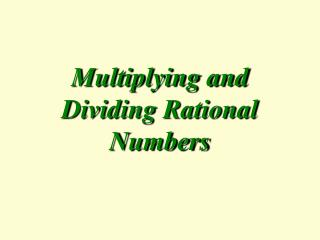DownloadDownload PresentationMultiplying and Dividing Rational Numbers

# Multiplying and Dividing Rational Numbers

Télécharger la présentation## Multiplying and Dividing Rational Numbers

- - - - - - - - - - - - - - - - - - - - - - - - - - - E N D - - - - - - - - - - - - - - - - - - - - - - - - - - -
##### Presentation Transcript

1. Multiplying and Dividing Rational Numbers

2. Rational Numbers • The term Rational Numbers refers to any number that can be written as a fraction. • This includes fractions that are reduced, fractions that can be reduced, mixed numbers, improper fractions, and even integers and whole numbers. • An integer, like 4, can be written as a fraction by putting the number 1 under it.

3. Multiplying Fractions • When multiplying fractions, they do NOT need to have a common denominator. • To multiply two (or more) fractions, multiply across, numerator by numerator and denominator by denominator. • If the answer can be simplified, then simplify it. • Example: • Example:

4. 1 1 Simplifying Diagonally • When multiplying fractions, we can simplify the fractions and also simplify diagonally. This isn’t necessary, but it can make the numbers smaller and keep you from simplifying at the end. • From the last slide: • An alternative: You do not have to simplify diagonally, it is just an option. If you are more comfortable, multiply across and simplify at the end.

5. 1 1 Mixed Numbers • To multiply mixed numbers, convert them to improper fractions first.

6. Sign Rules • Remember, when multiplying signed numbers... Positive * Positive = Positive. Negative * Negative = Positive. Positive * Negative = Negative.

7. Try These: Multiply Multiply the following fractions and mixed numbers:

8. Solutions: Multiply

9. 2 1 2 1 1 3 1 2 1 3 Solutions (alternative): Multiply Note: Problems 1, 2 and 4 could have been simplified before multiplying.

10. Change Operation. Flip 2nd Fraction. Dividing Fractions • When dividing fractions, they do NOT need to have a common denominator. • To divide two fractions, change the operation to multiply and take the reciprocal of the second fraction (flip the second fraction). Keep-Change-Change.

11. Dividing Fractions • Finish the problem by following the rules for multiplying fractions.

12. Try These: Divide • Divide the following fractions & mixed numbers:

13. Solutions: Divide